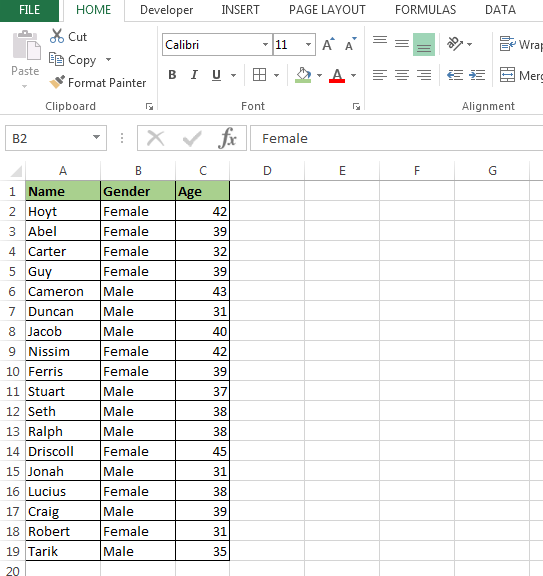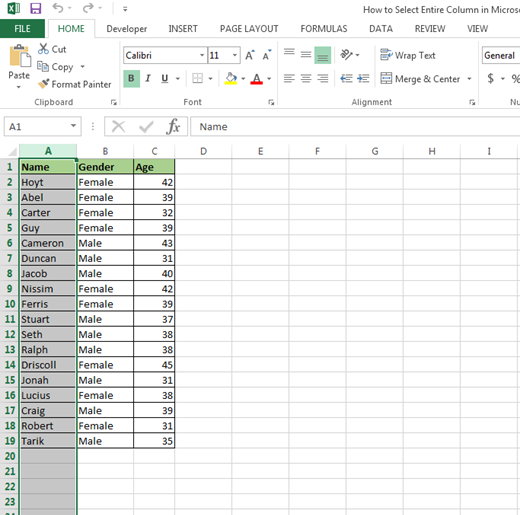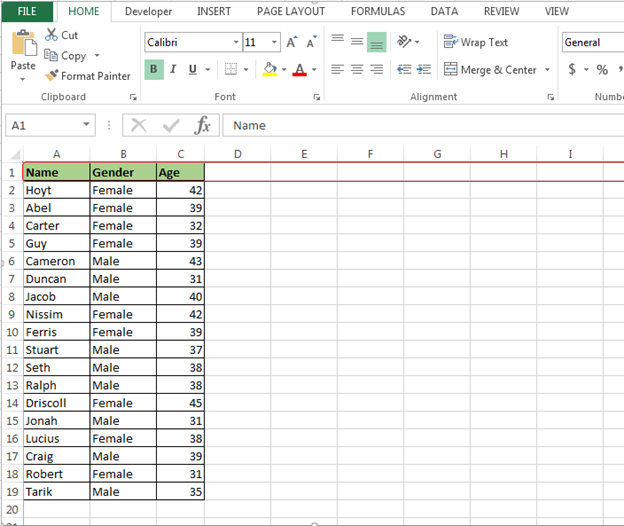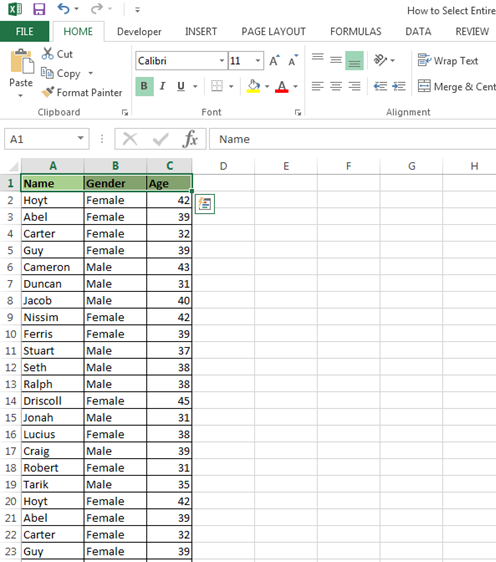# Copy Selection to a Database sheet using VBA in Microsoft Excel

When we write the VBA coding to save ourselves from the repetitive work, selection of data plays a very important role. So, in this article you will learn how to select the data by row, column and whole documents data through VBA coding in Microsoft Excel.

You’ll learn:

• How to select entire column?
• How to select entire row?
• How to select entire data?

How to select entire column through VBA?

We have data in the range A1:C19, in which column A contains Name, column B Gender and column C contains Age.To select the Entire column A in this data, follow below given steps and code:-

• Open VBA Page press the key Alt+F11
• Insert a module
• Write the below mentioned code:

```Sub Columnselect()

Range("A1").Entirecolumn.Select

End Sub
```

• Press the key F5 to run the Macro in Excel
• Column A will get selectedIf you want data should be selected till the last active cell to the column, follow below mentioned steps and code:-

• Write the below mentioned code:-

```Sub Columnselect()

lastrow = Worksheets("Sheet1").Cells(Rows.count, 1).End(xlUp).Row

Worksheets("Sheet1").Range("A1:A" & lastrow).Select

End Sub
```

Code Explanation:- First we will define that what will be last active cell in data, and then last row we will use to define the range in coding.

• Press the key F5 to run the Macro in Excel
• Column A1:A19 will get selected

In this way by using VBA, you can select the code to select the column till last active cell. When we don’t know what the last active cell is, we can use this code while writing the VBA code for the selection in Microsoft Excel.

How to select entire row through VBA?

We have data in the range A1:C19, in which column A contains Name, column B Gender and column C contains Age.To select the Entire row in this data follow below given steps and code:-

• Open VBA Page press the key Alt+F11
• Insert a module
• Write the below mentioned code:-

```Sub rowselect()

Range("A2").EntireRow.Select

End Sub
```

• Press the key F5 to run the Macro in Excel
• Column A will get selectedIf you want data should be selected till the last active cell to row follow below mentioned steps and code:-

• Write the below mentioned code:-

```Sub rowselect()

Dim lastcolumn As Long

lastcolumn = Worksheets("Sheet1").Cells(1, Columns.count).End(xlToLeft).Column

Worksheets("Sheet1").Range("A1", Cells(1, lastcolumn)).Select

End Sub
```

Code Explanation: In this code we had defined the last column first, then we will use that to define the range.

• Press F5 to run the Macro in Excel
• First row will get selected till the last active cell to the rowIn this way we can select the entire row or select the row till the last active cell in Microsoft Excel through VBA.

How to select entire data through VBA?

Let’s take an example and understand:-

We have data in the range A1:C19, in which column A contains Name, column B Gender and column C contains Age.To select the data from first cell to last active cell follow below given steps and code:-

• Open VBA Page press the key Alt+F11
• Insert a module
• Write the below mentioned code:

```Sub Selectionoflastcell()

Dim lastrow As Long, lastcolumn As Long

lastrow = Worksheets("Sheet1").Cells(Rows.count, 1).End(xlUp).Row

lastcolumn = Worksheets("Sheet1").Cells(1, Columns.count).End(xlToLeft).Column

Worksheets("Sheet1").Range("A1", Cells(lastrow, lastcolumn)).Select

End Sub
```

Code Explanation: First we have defined the Subject name of Code, then we have defined all the variables. Then we have defined what would be our last row and last column.

• Press the key F5 to run the Macro in Excel
• In the worksheet all data will get selected

Now, if you want to copy same data and paste it use below mentioned code:-

```Sub Selectionoflastcell()

Dim lastrow As Long, lastcolumn As Long

lastrow = Worksheets("Sheet1").Cells(Rows.count, 1).End(xlUp).Row

lastcolumn = Worksheets("Sheet1").Cells(1, Columns.count).End(xlToLeft).Column

Worksheets("Sheet1").Range("A1", Cells(lastrow, lastcolumn)).Copy Sheets("Sheet2").Range("A1")

End Sub
```

Run the code all data will get copied and pasted in sheet2.

This is the way we can write the macro for select the data from first cell to last active cell and then paste in other sheet in Microsoft Excel.